# Lewis structures

## What are Lewis Structures?

Gilbert Newton Lewis proposed that for a molecule to be stable its valence shell should be complete. Hydrogen, with 1 electron in an s orbital, needs only 1 electron to complete its valence shell. However, since carbon has 4 valence electrons, it will need to complete the 4 valence (s , px, py, pz) orbitals with 4 additional electrons, to reach a total of 8 valence electrons (octet rule). This electronic configuration is particularly stable and similar to that of a noble gas.

To visualize this, G.N. Lewis developed a representation system where valence electrons are indicated around atoms with dots () and a pair of electrons with a colon (:). It is still considered one of the most famous forms of structure and representation of organic molecules.For example, in the figure above, hydrogen, carbon, nitrogen, oxygen and fluorine atoms are depicted with their valence electrons (1, 4, 5, 5, 6 and 7, respectively), as well as Lewis structures for CH3F (fluoromethane) and HCONH2(formamide) molecules. Alternatively, the electrons can be represented with the symbols to visualize more clearly their «origin«.

In addition, to further simplify the structures, instead of dots, lines () are drawn to represent bonds and dots for non-bonding electrons (non-bonding electrons). In other texts such representations appear with lines only.

The following figure shows different forms of Lewis representation for the ClCCONH2 molecule. Regardless of how the electrons are drawn, they all show that oxygen has two unshared electron pairs and forms a double bond with carbon, nitrogen has only one unshared electron pair, and chlorine has three.To summarize, Lewis structures show, in a simple graphical form, the number and type of bonds in a molecule (connectivity), as well as the number of unshared electron pairs, or unpaired electrons.

## Formal charge

Formal charge is a concept in chemistry that is used to determine the distribution of electrons in a molecule or ion. It is a way of assigning charges to individual atoms in a molecule or ion, based on the number of valence electrons it has, and the number of electrons it shares with other atoms in the molecule.

Thus, the formal charge of an atom is the charge that atom would have if all the bonds present in that atom were pure covalent.
The formal charge on an atom is calculated by the following formula:
formal charge of an atom = (no. e valence) – (no. e non-bonded) – ½ (no. e bonded)
For example, the formal charge of the carbon atom is 0 in methane, and we calculate it as follows:The number of valence electrons of carbon is 4.
It has no unbonded electrons on carbon.
The bonded electrons of carbon are 8.
Therefore, the formal charge of carbon will be zero = (4) – (0) – ½(8) = 0.

## Resonance structures

Within the structure and representation of organic molecules, there are many that have particular properties due to a special electron density distribution. Among these are the aromatic compounds, for example benzene. Such compounds can be described by various Lewis structures, and are called resonant structures, and differ only in the distribution of their electrons (not that of the atoms). For example benzene can be represented, mainly, by two resonant structures shown in the figure.It should be noted that both describe benzene, being in reality a structure with intermediate characteristics (for example, the length of the C-C bonds would be intermediate between that of a single and a double bond). Resonant structures are usually indicated in parentheses and are related with a double-headed arrow, which should not be confused with systems in equilibrium, reactions, etc.

Furthermore, we should not change the nuclear positions and orientation of the molecule in such structures. The resonance hybrid would be a unique structure representing this situation.

The different resonant structures have a greater or lesser contribution to the resonance hybrid depending on the stability of each one of them (structures with lower energy contribute more), and such contribution can be qualitatively evaluated taking into account the following considerations:

a) The most stable resonant structure (that contributes the most) is the one with the highest number of covalent bonds.b) The most stable resonant structure (contributing more) is the one with the highest number of octets (2 and 1 in the previous case).
c) Uncharged structures are more stable (contribute more) than those with charge separation.d) When comparing two resonant structures with charge, the one with its negative charge on the more electronegative atom will be more stable.
e) When comparing two resonant structures with charge, the one with a minimum separation of charges of different sign will be more stable. Also, the structure with the same charge on adjacent atoms is more unstable.## Examples of Lewis structures

### Acetone molecule

Acetone has molecular formula CH3COCH3 and zero formal charge.

• First step: Choose the central atom. In this case, the carbonyl carbon atom (blue). Place all the other atoms around it by adding the valence electrons with the symbols “x” and “o”. Making sure that in contiguous atoms we have different types so as not to get confused.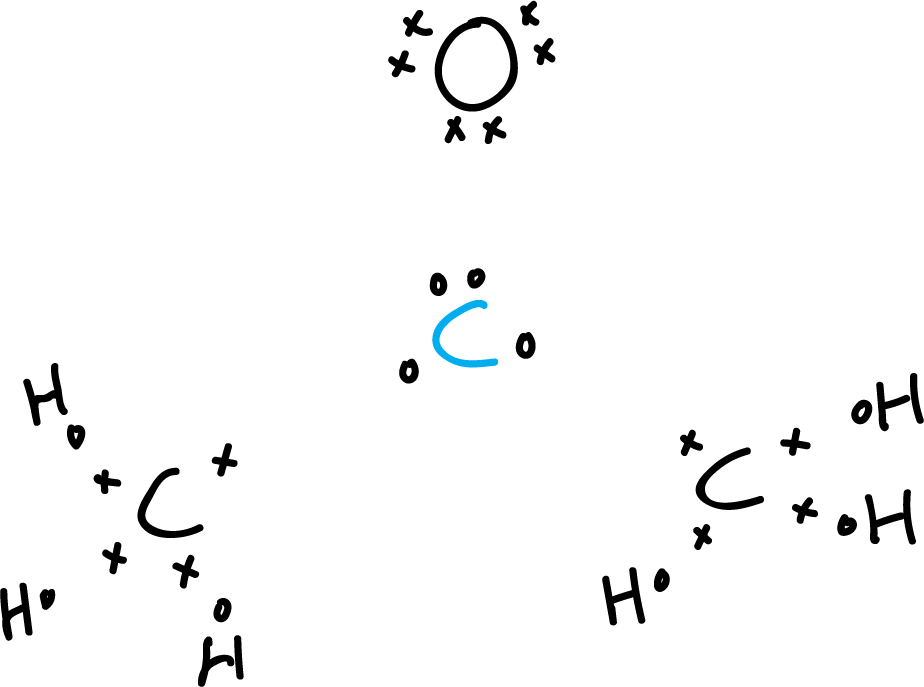• Second step: Count the valence electrons. The carbons present four valence electrons and
the oxygen presents 6, and the six hydrogens 1, each respectively.
This makes a total of (3 × 4) + (6 × 1) + (1 × 6)= 24.
Acetone has no net, extra or minus charge due to negative or positive ions.
Therefore, the total number of electrons is 24. We draw the resulting valence bonds (orange lines).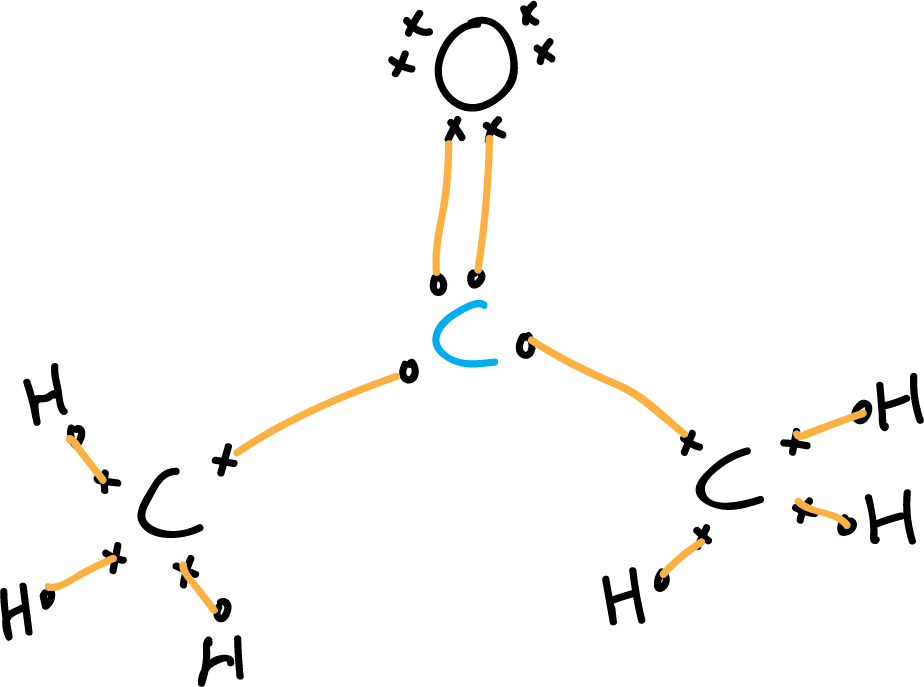• Third step: Locate the electron pairs and adjust to the octet rule.
The carbons are bonded to the central carbon (carbonyl carbon marked in blue).
We do the same with the nitrogen and with the hydrogens.
We look for the electrons needed to fulfill the octet rule.
As the four atoms (three carbons and one oxygen)
must fulfill it, the total number would be 4 x 8 = 32 electrons to fulfill the octet rule,
but we must subtract the 6 electrons of the hydrogens (32 – 6 = 26), so we are left with 26 electrons.
According to the previous calculations the number of bonding electrons
would be 26 – 24 = 1 corresponding to one more pair of the double bond of the carbonyl group. We draw the unbonded electron pairs (green lines).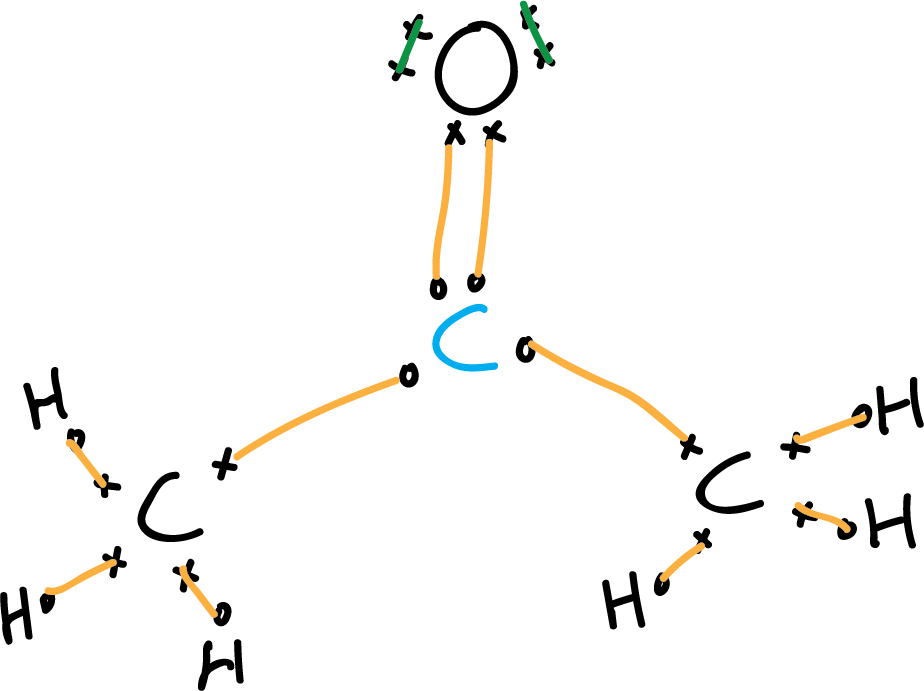• Fourth step: Non-bonding electrons (unshared electron pairs).
To locate the non-bonding electrons simply calculate the difference between the total valence electrons minus the bonding electrons: 32 – (26 + 2) = 4 electrons corresponding to 2 electron pairs, which are located under the premise of compliance with the octet rule are assigned to oxygen. (The drawing can be simplified by eliminating the electrons).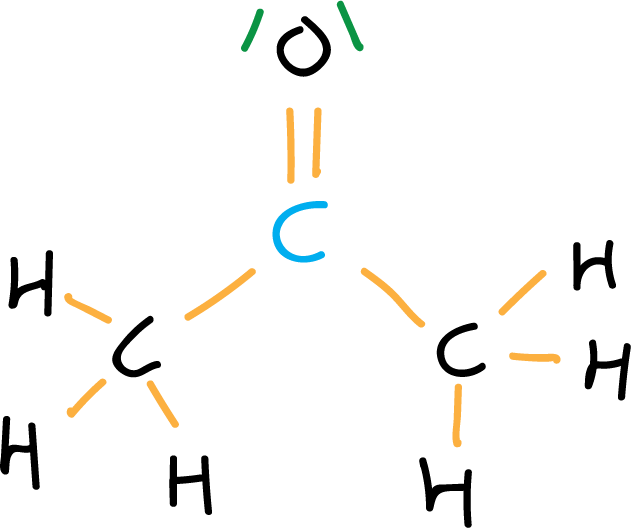• Fifth step: Formal charge. if formal charge exists, it is located on the corresponding atom. In this case the formal charge is zero. Generally, the representation of the acetone molecule is further simplified by eliminating the C-H bonds, and representing the electron pairs by dots.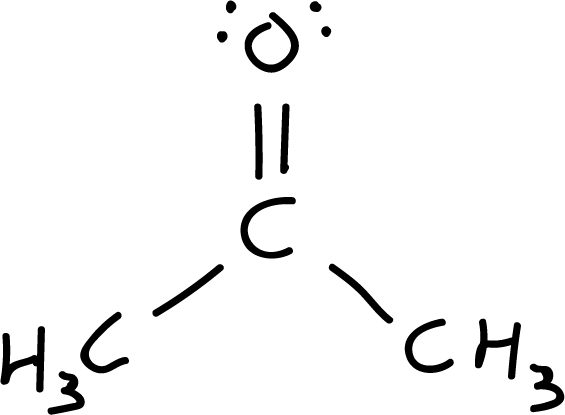• Step 6: Resonant structures. Resonant structures are explored by relocating charges and bonds. Starting from structure I, a pair of electrons is moved from the carbonyl double bond to the oxygen (more electronegative atom), resulting in the resonant structure II, which has a positive charge on the carbon and a negative charge on the oxygen (net charge is zero).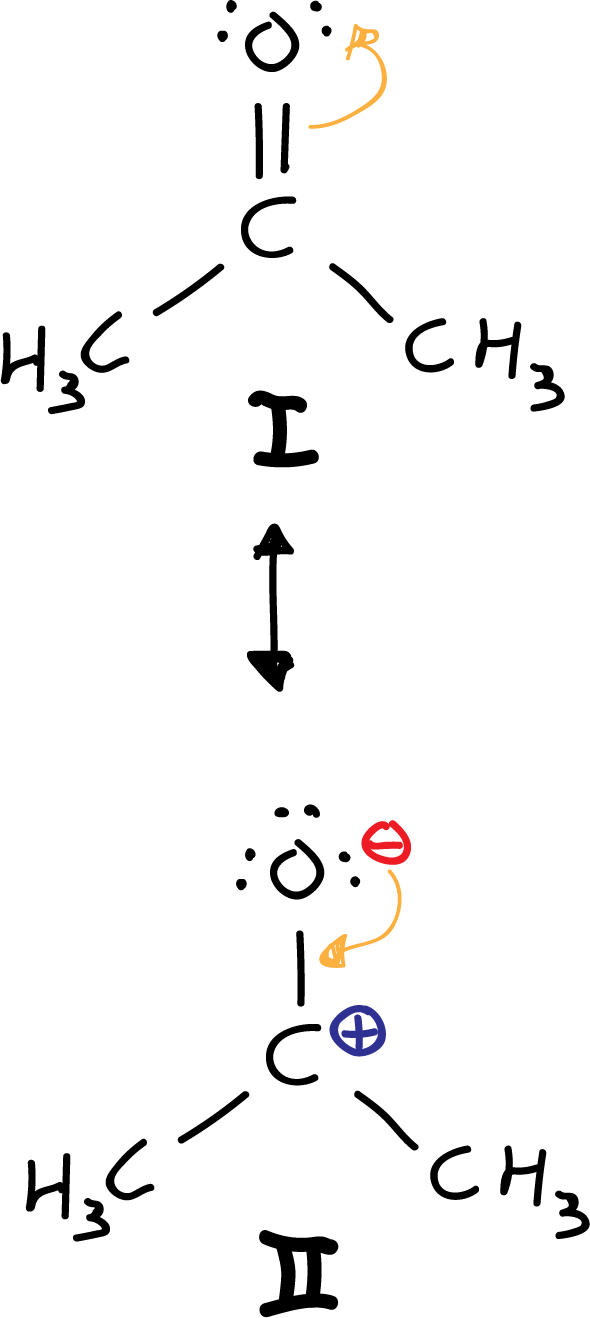Another option would be to move, from the I structure, a pair of electrons from the carbonyl double bond to the oxygen. However, because carbon is less electronegative (see electronegativity table) than oxygen, the III resonant structure is ruled out, because it results with an electronegative carbon and an electropositive oxygen.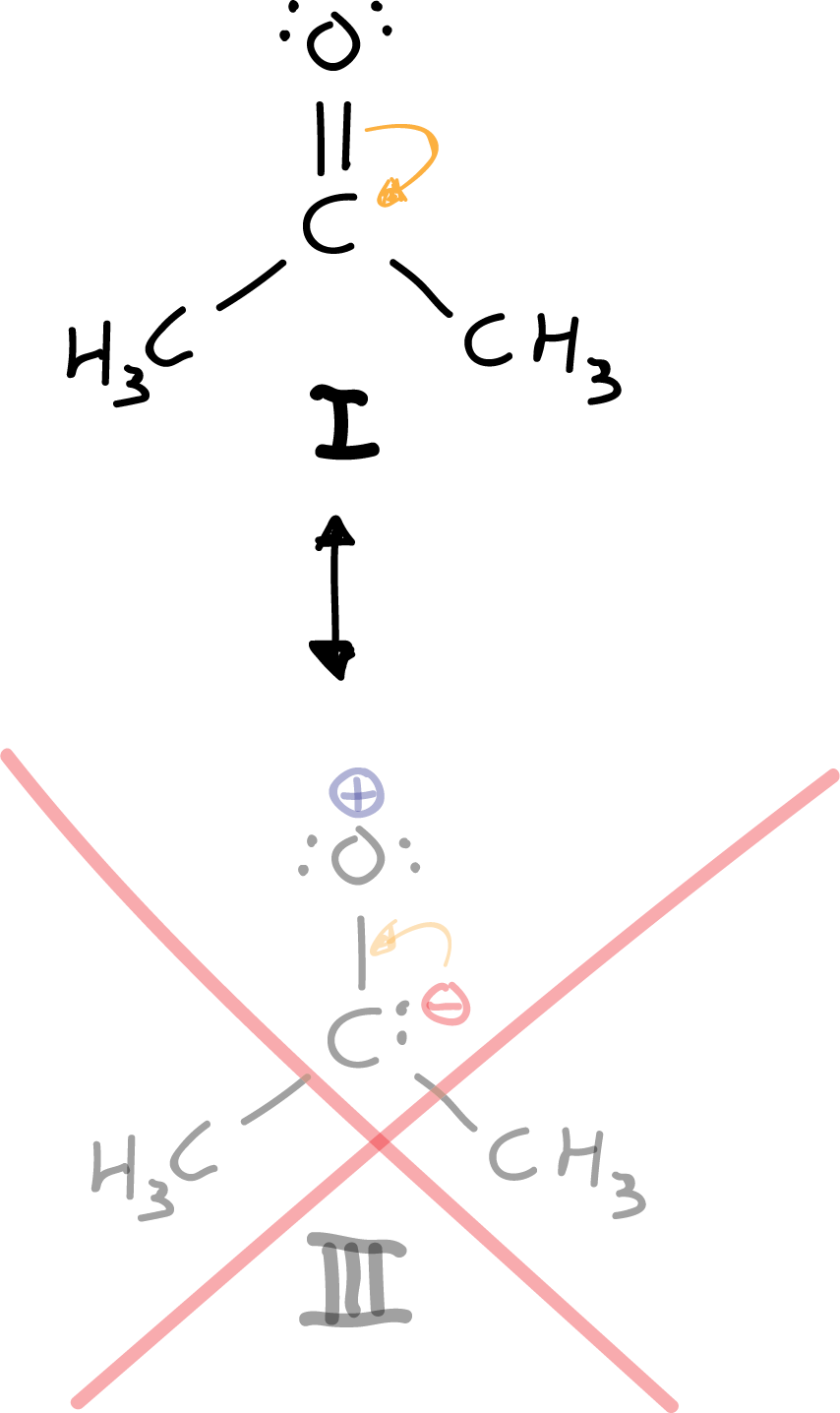There is only one resonant structure that complies with the octet rule, and that is structure I. Neither structure II nor IIIcomply with it, but III. In addition it is ruled out a priori because we are putting negative charge to an atom that is not the most electronegative of the molecule.

Shares